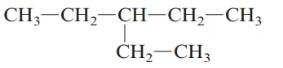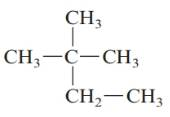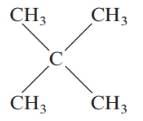Chapter 20, Problem 26QAP### Introductory Chemistry: A Foundati...

9th Edition
Steven S. Zumdahl + 1 other
ISBN: 9781337399425

#### Solutions

Chapter
Section### Introductory Chemistry: A Foundati...

9th Edition
Steven S. Zumdahl + 1 other
ISBN: 9781337399425
Textbook Problem
1 views

# . Give the systematic name for each of the following alkanes. a.b.c.d.Interpretation Introduction

Interpretation:

The systematic names of the given alkane should be determined.

Concept Introduction:

In order to give the IUPAC name to the branched-chain alkane following steps are followed:

1. The parent (longest) continuous carbon chain is identified.

2. The ending of the parent chain for alkane (-e) is -ane.

3. Name should be written in alphabetical order and numbering should be done in such a way that the substituent group gets lowest number.

4. Hyphen is used to connect the number to the name.

For number of carbons atoms in alkane chain, the prefix is given as:

Carbon-1meth

Carbon-2eth

Carbon-3prop

Carbon-4but

Carbon-5pent

Carbon-6hex

Carbon-7hept

Carbon-8oct

Carbon-9non

Carbon-10dec.

Explanation

The given structure is:

The parent chain in the given structure is pentane...

Interpretation Introduction

Interpretation:

The systematic names of the given alkane should be determined.

Concept Introduction:

In order to give the IUPAC name to the branched-chain alkane following steps are followed:

1. The parent (longest) continuous carbon chain is identified.

2. The ending of the parent chain for alkane (-e) is -ane.

3. Name should be written in alphabetical order and numbering should be done in such a way that the substituent group gets lowest number.

4. Hyphen is used to connect the number to the name.

For number of carbons atoms in alkane chain, the prefix is given as:

Carbon-1meth

Carbon-2eth

Carbon-3prop

Carbon-4but

Carbon-5pent

Carbon-6hex

Carbon-7hept

Carbon-8oct

Carbon-9non

Carbon-10dec.

Interpretation Introduction

Interpretation:

The systematic names of the given alkane should be determined.

Concept Introduction:

In order to give the IUPAC name to the branched-chain alkane following steps are followed:

1. The parent (longest) continuous carbon chain is identified.

2. The ending of the parent chain for alkane (-e) is -ane.

3. Name should be written in alphabetical order and numbering should be done in such a way that the substituent group gets lowest number.

4. Hyphen is used to connect the number to the name.

For number of carbons atoms in alkane chain, the prefix is given as:

Carbon-1meth

Carbon-2eth

Carbon-3prop

Carbon-4but

Carbon-5pent

Carbon-6hex

Carbon-7hept

Carbon-8oct

Carbon-9non

Carbon-10dec.

Interpretation Introduction

Interpretation:

The systematic names of the given alkane should be determined.

Concept Introduction:

In order to give the IUPAC name to the branched-chain alkane following steps are followed:

1. The parent (longest) continuous carbon chain is identified.

2. The ending of the parent chain for alkane (-e) is -ane.

3. Name should be written in alphabetical order and numbering should be done in such a way that the substituent group gets lowest number.

4. Hyphen is used to connect the number to the name.

For number of carbons atoms in alkane chain, the prefix is given as:

Carbon-1meth

Carbon-2eth

Carbon-3prop

Carbon-4but

Carbon-5pent

Carbon-6hex

Carbon-7hept

Carbon-8oct

Carbon-9non

Carbon-10dec.

### Still sussing out bartleby?

Check out a sample textbook solution.

See a sample solution

#### The Solution to Your Study Problems

Bartleby provides explanations to thousands of textbook problems written by our experts, many with advanced degrees!

Get Started